Courses

Previous Year Questions (2016-20): Momentum and Impulse | JEE Main Notes | Study Physics 35 Years JEE Main & Advanced Past year Papers - JEE

JEE: Previous Year Questions (2016-20): Momentum and Impulse | JEE Main Notes | Study Physics 35 Years JEE Main & Advanced Past year Papers - JEE

The document Previous Year Questions (2016-20): Momentum and Impulse | JEE Main Notes | Study Physics 35 Years JEE Main & Advanced Past year Papers - JEE is a part of the JEE Course Physics 35 Years JEE Main & Advanced Past year Papers.
All you need of JEE at this link: JEE

Q.1. A body A, of mass m = 0.1 kg has an initial velocity of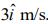It collides elastically with another body, B of the same mass which has an initial velocity of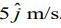. After collision, A moves with a velocity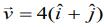. The energy of B after collision is written as ( x/10) J.  The value of x is _________.    (2020)
Ans.
(1)
Solution.

Given that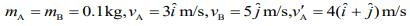Since, collision is elastic so by the conservation of linear momentum
Initial momentum = Final momentum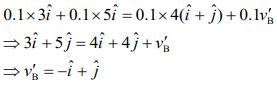Kinetic energy of B after collision is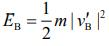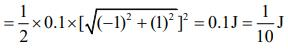Therefore, x = 1

Q.2. A particle of mass m is dropped from a height h above the ground. At the same time another particle of the same mass is thrown vertically upwards from the ground with a speed of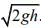If they collide head-on completely inelastically, the time for the combined mass to reach the ground, in units of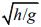is    (2020)
(1)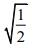(2)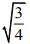(3) 1/2

(4)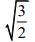Ans.
(4)
Solution.
Given that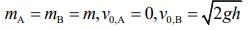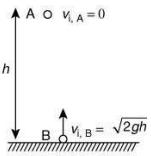Time for collision is given by t =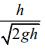Velocity before collision is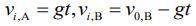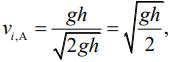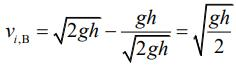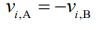From conservation of momentum, we have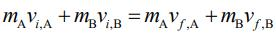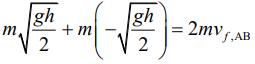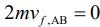So, height from ground is given by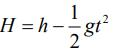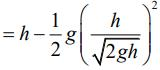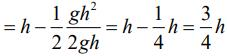Q. 3 A particle of mass m is projected with a speed u from the ground at an angle θ = π/3 w.r.t. horizontal (x-axis). When it has reached its maximum height, it collides completely inelastically with another particle of the same mass and velocity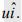. The horizontal distance covered by the combined mass before reaching the ground is    (2020)
(1)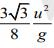(2)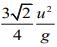(3)(4)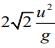Ans. (1)
Solution.

Given that initial speed u, angle of projection from ground θ = π/3.
Speed of particle at height point is given by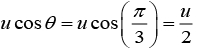Applying conservation of momentum, we have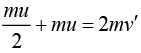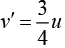Time of flight after collision is given by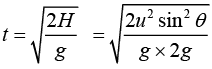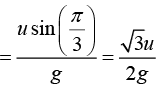Range after collision is given by R =v′t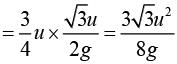Q 4. A particle is moving along a circular path with a constant speed of 10 m/s. What is the magnitude of the change in velocity of the particle, when it moves through an angle of 60° around the center of the circle?    (2019)
(1) 10√2 m/s
(2) 10√3 m/s
(3) 10 m/s
(4) zero
Ans:
(3)
Solution: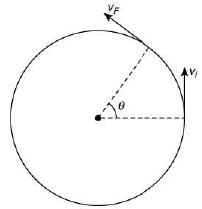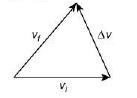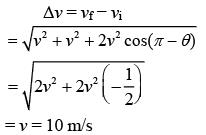Q 5. A particle of mass 20 g is released with an initial velocity 5 m/s along the curve from the point a, as shown in the figure. The point a is at height h from point B. The particle slides along the frictionless surface. When the particle reaches point B, its angular momentum about O will be (take g = 10 m/s2)    (2019)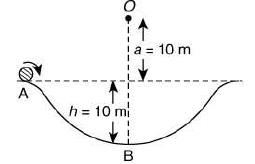(1) 2 kg·m2/s
(2) 8 kg·m2/s
(3) 6 kg·m2/ s
(4) 3 kg·m2/ s
Ans: (3)
Solution:
According to the conservation of energy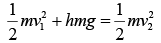Given, h = 10 m; v1 = 5 m; g = 10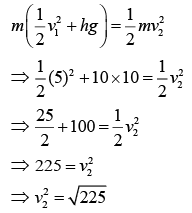Angular momentum at point B about point O is
L = mrv        (r = h + a)
= mv2(h + a)
= 20 × 10−3 × 15 × 20 = 6 kg m2/s

Q 6. A particle of mass m is moving along the side of a square of side ‘a’, with a uniform speed v in the x-y plane as shown in the figure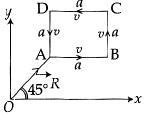Which of the following statements is false for the angular momentum L about the origin?      (2016)

(1)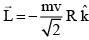when the particle is moving from A to B

(2)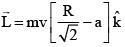when the particle is moving from C to D

(3)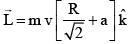when the particle is moving from B to C

(4)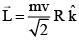when the particle is moving from D to A
Ans:
(2,4)
Solution:
Along CD, ⊥ distance from line of motion =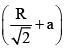∴ Magnitude of angular momentum =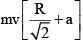Hence (2) is incorrect.
In option (4) the direction of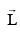is incorrect.

Q 7. In the figure shown/ ABC is a uniform wire. If centre of mass wire lies vertically below point A, then    (2016)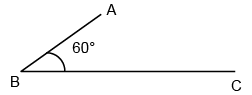1)1.85
(2) 1.5
(3) 3
(4) 1.37
Ans:
(4)
Solution: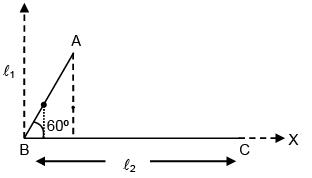If CM lies vertically below A ⇒ as per choose coordinate axis in x- coordinate is equal to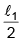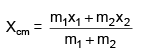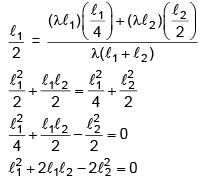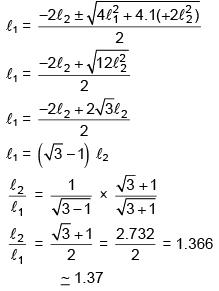The document Previous Year Questions (2016-20): Momentum and Impulse | JEE Main Notes | Study Physics 35 Years JEE Main & Advanced Past year Papers - JEE is a part of the JEE Course Physics 35 Years JEE Main & Advanced Past year Papers.
All you need of JEE at this link: JEEUse Code STAYHOME200 and get INR 200 additional OFF Use Coupon Code

Top Courses for JEE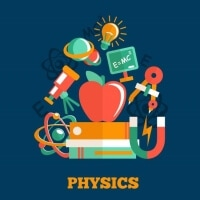Physics 35 Years JEE Main & Advanced Past year Papers

88 docs|49 tests

Top Courses for JEETrack your progress, build streaks, highlight & save important lessons and more!

,

,

,

,

,

,

,

,

,

,

,

,

,

,

,

,

,

,

,

,

,

;